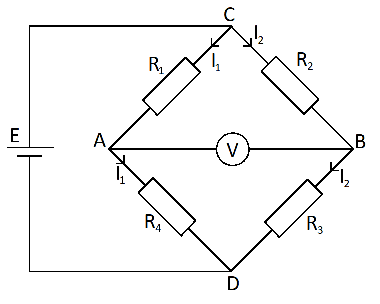# Proof of Wheatstone bridge equation

• whatdoido

## Homework Statement

Prove the following equation:

## \Delta U=\frac {R_1R_4}{(R_1+R_4)^2}(\frac {\Delta R_1}{R_1}-\frac {\Delta R_2}{R_2}+\frac{\Delta R_3}{R_3}-\frac{\Delta R_4}{R_4})E##

This is used in Wheatstone bridge[/B]
U=RI

## The Attempt at a Solution

This has been a real head-scratcher

Two voltage dividers can be found for starters. Voltage's direction is assumed to be clockwise

##V_{in1}=I_2(R_2+R_3)##

##I_2=\frac{V_{in1}}{R_2+R_3}##

##V_{out1}=I_2R_3##

##V_{out1}=V_{in1}\frac{R_3}{R_2+R_3}##

Similarly:

##V_{out2}=V_{in1}\frac{R_4}{R_1+R_4}##

##V_G## is voltage between A and B

##V_{out1}-V_{out2}=V_G##

##V_{in1}\frac{R_3}{R_2+R_3}-V_{in1}\frac{R_4}{R_1+R_4}=V_G##

##V_{in1}(\frac{R_3}{R_2+R_3}-\frac{R_4}{R_1+R_4})=V_G##

##V_{in1}=E##

##V_G=\Delta U## so then

##E(\frac{R_3}{R_2+R_3}-\frac{R_4}{R_1+R_4})=\Delta U##

I have calculated voltages in different circuits and tried to think this problem in different ways, but the real problem is that how is ##\Delta R_i## inserted into equations. Assumption goes that it is added by ##R_i+\Delta R_i##. Maybe that is incorrect?

Help is very much appreciated!

edit: Misspelling corrected

Also particularizing that ##\Delta R_i## is a change in one resistance

#### Attachments

Last edited:

that ##\Delta R_i## is a change in one resistance
Which suggests that the Δ in ΔU refers to the consequent change in U, not to the potential difference between A and B at a given set of R values.

Which suggests that the Δ in ΔU refers to the consequent change in U, not to the potential difference between A and B at a given set of R values.
Yes that is true, ##\Delta U## is zero before the change of resistances.

Yes that is true, ##\Delta U## is zero before the change of resistances.
So this equation:
##E(\frac{R_3}{R_2+R_3}-\frac{R_4}{R_1+R_4})=\Delta U##
##E(\frac{R_3}{R_2+R_3}-\frac{R_4}{R_1+R_4})=U##
and you need a different expression for ##\Delta U##.

Right now I'm trying to figure out why this would not be possible:

##E(\frac{R_3+\Delta R_3}{R_2+\Delta R_2+R_3+\Delta R_3}-\frac{R_4+\Delta R_4}{R_1+\Delta R_1+R_4+\Delta R_4})=\Delta U##

I can simplify it a bit, but is this the right way to go

Right now I'm trying to figure out why this would not be possible:

##E(\frac{R_3+\Delta R_3}{R_2+\Delta R_2+R_3+\Delta R_3}-\frac{R_4+\Delta R_4}{R_1+\Delta R_1+R_4+\Delta R_4})=\Delta U##

I can simplify it a bit, but is this the right way to go
I do not see how you get that. It looks wrong.
You have an equation for U (second eqn in post #4). Write out the corresponding eqn for U+ΔU.

I do not see how you get that. It looks wrong.
You have an equation for U (second eqn in post #4). Write out the corresponding eqn for U+ΔU.
Now that I thought about it, simply adding the change does not make so much sense.

But then I got an idea to take partial derivates since it is about change. Adding those partial derivates together should give the overall change in voltage.

##U=E(\frac{R_3}{R_2+R_3}-\frac{R_4}{R_1+R_4})##

Seems like marking ##U## as ##U_{BA}## is needed since I took potential difference with ##V_{out1}-V_{out2}=V_G##

##U_{BA}=(\frac{R_3}{R_2+R_3}-\frac{R_4}{R_1+R_4})E##

This should be legal: ##\Delta U_{BA}=dU_{BA}##

Thus ##dU_{BA}=\frac {\partial} {\partial R_1}U_{BA}\Delta R_1+\frac {\partial} {\partial R_2}U_{BA}\Delta R_2+\frac {\partial} {\partial R_3}U_{BA}\Delta R_3+\frac {\partial} {\partial R_4}U_{BA}\Delta R_4##

Solving partial derivates each:

##\frac {\partial} {\partial R_1}U_{BA}\Delta R_1=\frac {\partial} {\partial R_1}(\frac{R_3}{R_2+R_3}-\frac{R_4}{R_1+R_4})E\Delta R_1=-\frac {R_4}{(R_1+R_4)^2}E\Delta R_1##

##\frac {\partial} {\partial R_2}U_{BA}\Delta R_2=\frac {\partial} {\partial R_2}(\frac{R_3}{R_2+R_3}-\frac{R_4}{R_1+R_4})E\Delta R_2=\frac{R_3}{(R_2+R_3)^2}E\Delta R_2##

##\frac {\partial} {\partial R_3}U_{BA}\Delta R_3=\frac {\partial} {\partial R_3}(\frac{R_3}{R_2+R_3}-\frac{R_4}{R_1+R_4})E\Delta R_3=-\frac{R_2}{(R_2+R_3)^2}E\Delta R_3##

##\frac {\partial} {\partial R_4}U_{BA}\Delta R_4=\frac {\partial} {\partial R_4}(\frac{R_3}{R_2+R_3}-\frac{R_4}{R_1+R_4})E\Delta R_4=\frac{R_1}{(R_1+R_4)^2}E\Delta R_4##

##dU_{BA}=-\frac {R_4}{(R_1+R_4)^2}E\Delta R_1+\frac{R_3}{(R_2+R_3)^2}E\Delta R_2-\frac{R_2}{(R_2+R_3)^2}E\Delta R_3+\frac{R_1}{(R_1+R_4)^2}E\Delta R_4##

##dU_{BA}=(-\frac{R_4}{R_1(1+\frac{R_4}{R_1})^2}\frac{\Delta R_1}{R_1}+\frac{R_3}{R_2(1+\frac{R_4}{R_1})^2}\frac{\Delta R_2}{R_2}-\frac{R_2}{R_3(1+\frac{R_1}{R_4})^2}\frac{\Delta R_3}{R_3}+\frac{R_1}{R_4(1+\frac{R_1}{R_4})^2}\frac{\Delta R_4}{R_4})E##

I just kept playing with the identity ##\frac{R_2}{R_3}=\frac{R_1}{R_4}## and I got:

##dU_{BA}=\frac{R_1R_4}{(R_1+R_4)^2}(-\frac{\Delta R_1}{R_1}+\frac{\Delta R_2}{R_2}-\frac{\Delta R_3}{R_3}+\frac{\Delta R_4}{R_4})E##

It has wrong signs because of ##V_{out1}-V_{out2}=V_G##

So I think the equation is about ##U_{AB}##

##\Delta U_{AB}=dU_{AB}=-dU_{BA}=-\frac{R_1R_4}{(R_1+R_4)^2}(-\frac{\Delta R_1}{R_1}+\frac{\Delta R_2}{R_2}-\frac{\Delta R_3}{R_3}+\frac{\Delta R_4}{R_4})E##

##=\frac{R_1R_4}{(R_1+R_4)^2}(\frac{\Delta R_1}{R_1}-\frac{\Delta R_2}{R_2}+\frac{\Delta R_3}{R_3}-\frac{\Delta R_4}{R_4})E##

Looks good.